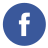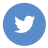### Counting Results in an Array

javascript

To count the number of results in an array in JavaScript, you can use the length property of the array. Here’s an example:

``````var myArray = ["apple", "banana", "orange", "grape"];

var numResults = myArray.length;

console.log("There are " + numResults + " results in the array.");``````

In this example, we have an array called myArray that contains four items. We can use the length property to get the number of items in the array, and assign the result to a variable called numResults. Finally, we use console.log() to output a message that displays the number of results in the array.

Note that the length property returns the total number of items in the array, regardless of whether they meet a certain criteria or not. If you only want to count items that meet a specific condition, you can use a loop to iterate through the array and count the items that match the condition. For example:

``````var myArray = [1, 2, 3, 4, 5, 6, 7, 8, 9, 10];

var count = 0;

for (var i = 0; i < myArray.length; i++) {
if (myArray[i] % 2 === 0) { // Count even numbers
count++;
}
}

console.log("There are " + count + " even numbers in the array.");``````

In this example, we loop through the array and increment a counter variable (count) each time we encounter an even number. At the end of the loop, we output a message that displays the count of even numbers.